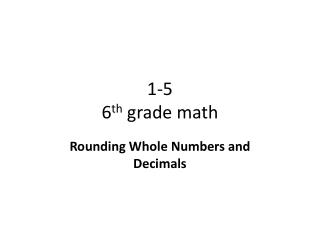Download Presentation# 1-5 6 th grade math - PowerPoint PPT PresentationDownload Presentation## 1-5 6 th grade math

- - - - - - - - - - - - - - - - - - - - - - - - - - - E N D - - - - - - - - - - - - - - - - - - - - - - - - - - -
##### Presentation Transcript

1. 1-56th grade math Rounding Whole Numbers and Decimals

2. Objective • To estimate and round whole numbers and decimals. • Why? To help you understand and approximate rounding and estimating of whole numbers and decimals.

3. California State Standards NS 1.1 (Gr. 5): Manipulate very large (e.g., millions) and very small (e.g., thousandths) numbers. MR 1.0: Make decisions about how to approach problems.

4. Vocabulary • Rounding • To change a number to the nearest 10, 100, 100, tenth, hundredth, thousandth, etc. • Remember any number whose ones digit is between 0 and 4 will not be rounded. But a digits whose number is between 5 and 9 will be rounded up. • 44 = 40; 4.67 = 5 or 4.7 • Estimation To round a number

5. How to Round Whole Numbers and Decimals 1) Read the directions for what place value ‘to round to’. 2) Find the place being asked and underline it. 3) Look to the digit to the right. 4a) If the digit is less than 5, keep the number the same. 4b) If the digit is more than 5, round the number up. 5) Keep all the numbers to the left the same, change or keep the asked digit, change all other digits to zeros (0’s). Round to nearest tenth. 14.77 = 14.77 = 14.80 Round to the nearest hundred. 1,639 = 1,639 = 1,600

6. Try It! Round 2,593.6781 to nearest places. • Nearest hundred • Nearest thousandths • Nearest tenth • Honolulu’s average rainfall to nearest ten inches. • 2,593.6781 = 2,593.6781 = 2,600.0000 2) 2,593.6781 = 2,593.6781 = 2,593.6780 3) 2,593.6781 = 2,593.6781 = 2,593.7000 4) 22.02 = 20.00 inches

7. Objective Review • You can now use To estimate and round whole numbers and decimals. • Why? You can now approximate whole numbers and decimals. This will help you when you are checking for reasonableness. To round decimals to a given place, follow the same steps as for rounding whole numbers. Pay attention to the given place and the place to its right in order to round.

8. Independent Practice • Complete problems 5-17 • Copy original problem first. • Show all work! • If time, complete Mixed Review: 18-23 • If still more time, work on Accelerated Math.